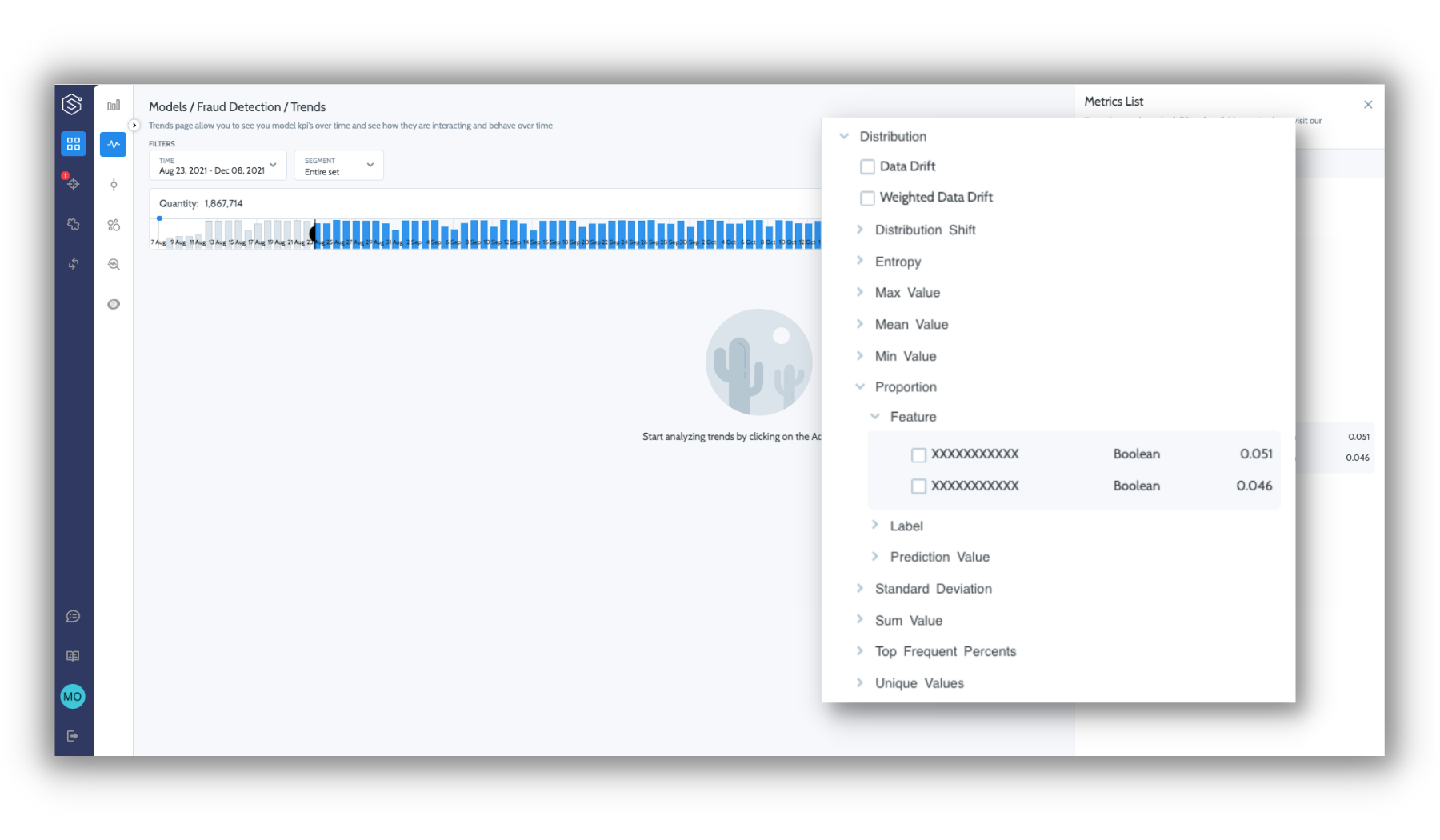# Distribution metrics

Statistical measures for any data entity throughout your ML processStatistical property metrics measured on the entity level are divided according to data entity format type:

Metric

Categorical

Numeric

Boolean

Top frequent percents
Frequency of the mode value.

Unique values
The number of unique values.

Entropy
Calculation of the entropy on the distribution of categorical entities.

Min value
The lowest value.

Max value
The highest value.

Sum value
The sum of values.

Mean value
The average value.

Standard Deviation
The measure of the amount of variation in a numeric entity.

Proportion
Percent of positive value.

#### Feature Importance

Superwise measures the effect a feature has on a model. Superwise calculates feature importance using SHAP values during baseline creation, or you can set this manually while configuring the schema.

#### Distribution shift & drift metrics:

• Distribution shift
How different the distribution in the selected data is from the baseline over time. The scale ranges from 0-100, where 0 indicates identical distribution, and 100 indicates orthogonal distribution.

• Input drift
The average distribution shifts across all features.